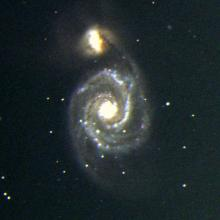# Equation for limiting magnitude of CCD camera?

2 posts / 0 new
CrossoverManiacEquation for limiting magnitude of CCD camera?

Also, as explained above, there are different factors to be considered for electronic imaging. In particular, the t in your formula would need to be replaced by square root of t because electronic noise increases with time as well as the signal from the star.

Thus a formula like this one would be more accurate:

LM = M + 2.5 * log10 (d * d * sqrt(t))

ie

LM = M + 5*log10(d) +1.25*log10(t)

For d in metres and t in seconds the value of M would be around 15 to 17 depending on the quality of the CCD camera used. This assumes that star size is atmosphere limited to about 2 arcsec diameter and that camera noise is greater than background sky brightness.

When star size is telescope resolution limited the equation would become:

LM = M + 10*log10(d) +1.25*log10(t)

and the value of M would be greater by about 3 magnitudes, ie a value 18 to 20.
For a practical telescope, the limiting magnitude will be between the values given by these 2 formulae.

I have been using the telescope resolution limited equation to get an idea of minimum apparent brightness of a star that would show up on .  However, there is the issue of defocusing, especially for photometry with a DSLR which, according to the AAVSO guide, the camera lens or telescope has to be defocused until the starlight from each star is at least eight pixels across for accurate photometry.  So, should M and t be reduced to represent the same amount of starlight falling on the CCD sensor but only across a larger number of pixels thus appearing fainter without extending the exposure time for each sub if the equation should be used at all?

RHM
Try an exposure calculator

It might be more useful to use one of the many CCD signal-to-noise calculators which you can find on-line.  These programs will take a bunch of information (diameter of telescope, sky brightness, CCD quantum efficiency and readnoise, etc., etc.) and compute the signal-to-noise ratio for a star within some given exposure time.  There are so many factors to consider that doing the arithmetic yourself can be a pain.

I wrote one simple calculator myself,

which might be enough for you.  If not, search for "CCD signal-to-noise calculator" and find one that works better.